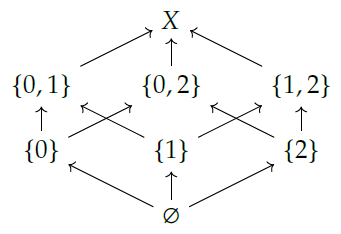Christopher wrote:

> The \$$\mathbf{Bool} \times \mathbf{Bool} \$$ interpretation seems to extend nicely to let one talk about \$$S\$$ indexed preorders as categories enriched in the space of (monotonic?) functions from \$$S\$$ to \$$\mathbf{Bool}\$$.

Yes! You didn't say what \$$S\$$ is, but if it's just a set we don't need "monotonic" functions from \$$S\$$ to \$$\mathbf{Bool}\$$; we can just look at _all_ functions from \$$S\$$ to \$$\mathbf{Bool}\$$. This is called \$$\mathbf{Bool}^S\$$, because you can also think of it as a product of copies of \$$\mathbf{Bool}\$$, one for each element of \$$S\$$:

$\prod_{s \in S} \mathbf{Bool} .$

\$$\mathbf{Bool}^S\$$ becomes a symmetric monoidal poset in an obvious way, and an \$$\mathbf{Bool}^S\$$-category is the same as a set \$$X\$$ with a bunch of different preorders, one for each element of \$$S\$$.

The "obvious way": given \$$f, g : S \to \mathbf{Bool}\$$, we say \$$f \le g\$$ iff

$f(s) \le g(s) \textrm{ for all } s \in S .$

This makes \$$\mathbf{Bool}^S\$$ into a poset, and then we make it into a symmetric monoidal poset using \$$\wedge \$$.

But in fact, the poset \$$\mathbf{Bool}^S\$$ is isomorphic to \$$P(S)\$$, the power set of \$$S\$$, made into a poset using \$$\subseteq\$$. It's easiest to understand this by looking at the case where \$$S\$$ has 3 elements. Then \$$P(S)\$$ is a cube:and you can see it's isomorphic to \$$\mathbf{Bool}^S \cong \mathbf{Bool} \times \mathbf{Bool} \times \mathbf{Bool} \$$. (Sorry, this picture calls \$$S\$$ "\$$X\$$".)

It's also interesting to think about what happens when \$$S\$$ is a _preorder_, and we look at _monotone_ maps \$$f : S \to \mathbf{Bool}\$$.